# Proposing a reaction rate mechanism

Gold Member

## Homework Statement

The rate law for the reaction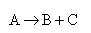is found from experiment to be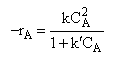Suggest a mechanism consistent with the rate law.

## The Attempt at a Solution

Here is the webpage with the solution
http://www.umich.edu/~elements/07chap/frames.htm

Here is the solution
Rate Laws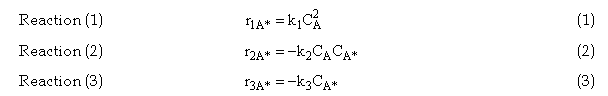Relative Rates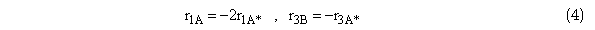Net Rates: Rate of Formation of Product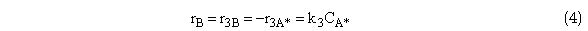Need to find an expression for CA* because we cannot easily measure the concentration of A*, use PSSH to solve for CA*.Solving for CA*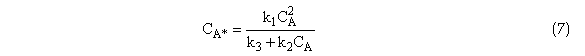Substituting for CA* in Equation (4) the rate of formation of B is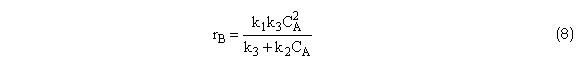Relative rates overall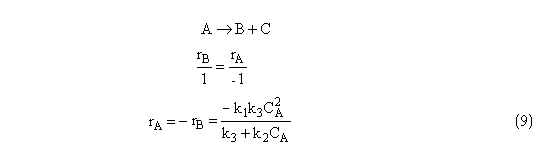My question is, why do they go about finding the rate law for B from the original reaction, instead of by steps. The way I thought to do this was to find ##r_{A}## from the elementary steps, exactly the same way they found ##r_{A^{*}}##.

##r_{1A} = -k_{1}C_{A}^2##
##r_{2A} = -k_{2}C_{A}C_{A^*}##
##r_{A} = r_{1A} + r_{2A}##

However, there is some complication with ##r_{A2}##. Since the reaction is ##A^* + A \rightarrow A + A##, How can I do the reaction rate of A assuming irreversible? I don't know if it should be ##r_{A2} = -k_{2}C_{A}C_{A^*}## or ##r_{A2} = -k_{2}C_{A}C_{A^*} + k_{2}C_{A}^2##

Last edited:

epenguin
Homework Helper
Gold Member
Sorry I cannot find the reaction, the proposed mechanism, the meaning of your symbols, and the experimental facts alluded to in your link. It would probably be best if you copied them to here. Though it looks like a mechanism of bimolecular collision followed by activation, which can be followed either by reversion/dissociation or onward retraction. But I'd need to be guessing what the problem is a bit less.

Gold Member
The problem statement is written as is. The purpose of this problem is to illustrate some basic rules of thumb for using a rate law given to determine the mechanism. These are the rules of thumb given in the textbook.

http://www.umich.edu/~elements/07chap/frames.htm

#### Attachments

Last edited:
epenguin
Homework Helper
Gold Member
Please state what is the hypothetical mechanism you are having trouble with. I could probably deduce what it probably is but this is unreasonable. You set out a series of equations, and after a bit I see you are using inconsistent terminology and your r1A is the same thing as rA1 etc, please correct. It now looks that there is a collisional activating step, but also a collisional deactivating step? (If so, at low concentrations the rate depends on collision frequency and the activated molecules react onwards because a second deactivating collison is rare, so overall rate is second-order. At high concentration most of the activated molecules are deactivated before they can react onwards, their concentration still increases with increasing A, but only linearly.)

Maybe your problem is only at the end you seem to have formulated two reactions as going the same way when they are opposed, and there is a - that should be a +, a sum that should be a difference?

Another guess - PSSH means something steady-state hypothesis?

Last edited:
Gold Member
Ok, so I fixed the part about the ordering of ##r_{1A}## vs. ##r_{A1}##, that was just a memory lapse. Apparently, the way to figure out the mechanism to a reaction is to memorize the table I have in my 2nd post. My problem is not memorizing the table. My issue is how they went on to find ##r_{A}## from their proposed mechanism. The proposed mechanism is

(1) ##A + A \xrightarrow {k_{1}} A^{*} + A## ##\hspace{0.5 in} r_{1A^{*}} = k_{1}C_{A}^2##
(2) ##A^{*} + A \xrightarrow {k_{2}} A + A## ##\hspace{0.5 in} r_{2A^{*}} = -k_{2}C_{A^{*}}C_{A}##
(3) ##A^{*} \xrightarrow {k_{3}} B + C ## ##\hspace{0.875 in} r_{3A^{*}} = -k_{3}C_{A^{*}}##

So then they sum ##r_{A^{*}} = r_{1A^{*}} + r_{2A^{*}} + r_{3A^{*}}##

Using the pseudo-steady state hypothesis, they say ##r_{A^{*}} = 0## and find an expression for ##C_{A^{*}}##. However, why is this step not repeated in finding ##r_{A}##? When they find ##r_{A}##, they simply say ##r_{B} = k_{3}C_{A^{*}}##, then substitute in ##C_{A^{*}}## found from ##r_{A^{*}}##, then using the original reaction ##A \rightarrow B + C##, they say ##r_{A} = -r_{B}##.

My proposal is to do exactly what they did for ##r_{A^{*}}##. I have an issue with reaction (1). How would you write ##r_{1A}## for that?

##r_{1A} =- k_{1} C_{A}^2##, or maybe it would be ##r_{1A} = -k_{1}C_{A}^2 + k_{1}C_{A}C_{A^{*}}##, or even perhaps something else? How do you write an elementary rate law with respect to a given species when the species is both a product and reactant, and the reaction is not reversible?

This issue is not relevant with respect to ##A^{*}## because that intermediate species is always either solely a reactant or solely a product, never both simultaneously in any of the 3 reaction steps.

Last edited:
epenguin
Homework Helper
Gold Member
OK, re my comment about + and -, sum and difference, I see they have put signs into your equations 1, 2, 3 and so your sum is an algebraic sum, which I think is slightly unusual presentation but OK now I know. In words the rate of change of [A*] is its rate of formation minus its rate of disappearance via two processes.

For your question check back to what they say about the PSSH. Assume that [A*] is small compared with [A]. Then
([A] + [B ]) is constant is just conservation of mass for that reaction, and rA = - rB is just saying the same thing (a molecule of A is converted into one of B).

B is created in a unimolecular elementary irreversible reaction from A*, step (3) and Eq. 3 is the physical consequence of this first-order process.

Why not do for rA what they did for rA* and equate it to 0? You notice if we do that we have no kinetics and no problem, that it would be saying A is not undergoing any reaction? A and B are at the ends of the scheme, A* is in the middle. Think of it like say a despatcher who sends a large amount from A on to B but in his own warehouse has only a small amount at any time. Because it is small the change of absolute amounts are small, it is A and B that are changing a lot.

Re notation I reccomend you where possible to write double subscripts for rates and rate constants telling what into what, and square molarity brackets [A] etc. which are hardly more cumbersome than subscripts like CA etc.* That way when you see a formula kA*B[A*] you and a reader instantly know what it refers to , instead of having to say, ah k3, now let's see, what was that? Or often species are labeled with numbers 1, 2, 3,... and in your mechanism you'd have k12, k21 and k23. It'll save you some errors and confusions,. Some journals were starting to reccomend or require this for publications when I was last following them much.

* a bit tricky to do in this forum as B inside [ ] gets read as instruction for bold text!

Last edited:
•gfd43tg
Gold Member
Alright thank you, I'm not sure why you are thinking that these reactions are unusual. The + and - dictate whether the concentration of the species is increasing or decreasing. The notation common in chemical engineering is ##C_{A}##, rather than ##[A]##, so I just follow the convention in my discipline.